# Frequency and time-frequency sensors analysis¶

The objective is to show you how to explore the spectral content of your data (frequency and time-frequency). Here we’ll work on Epochs.

We will use this dataset: Somatosensory. It contains so-called event related synchronizations (ERS) / desynchronizations (ERD) in the beta band.

# Authors: Alexandre Gramfort <alexandre.gramfort@inria.fr>
#          Stefan Appelhoff <stefan.appelhoff@mailbox.org>
#          Richard Höchenberger <richard.hoechenberger@gmail.com>
#
import os.path as op

import numpy as np
import matplotlib.pyplot as plt

import mne
from mne.time_frequency import tfr_morlet, psd_multitaper, psd_welch
from mne.datasets import somato


Set parameters

data_path = somato.data_path()
subject = '01'
raw_fname = op.join(data_path, 'sub-{}'.format(subject), 'meg',

# Setup for reading the raw data
events = mne.find_events(raw, stim_channel='STI 014')

picks = mne.pick_types(raw.info, meg='grad', eeg=False, eog=True, stim=False)

# Construct Epochs
event_id, tmin, tmax = 1, -1., 3.
baseline = (None, 0)
epochs = mne.Epochs(raw, events, event_id, tmin, tmax, picks=picks,

epochs.resample(200., npad='auto')  # resample to reduce computation time


Out:

Opening raw data file /home/circleci/mne_data/MNE-somato-data/sub-01/meg/sub-01_task-somato_meg.fif...
Range : 237600 ... 506999 =    791.189 ...  1688.266 secs
111 events found
Event IDs: 
111 matching events found
Applying baseline correction (mode: mean)
0 projection items activated
Rejecting  epoch based on EOG : ['EOG 061']
Rejecting  epoch based on EOG : ['EOG 061']
Rejecting  epoch based on EOG : ['EOG 061']


## Frequency analysis¶

We start by exploring the frequence content of our epochs.

Let’s first check out all channel types by averaging across epochs.

epochs.plot_psd(fmin=2., fmax=40., average=True, spatial_colors=False)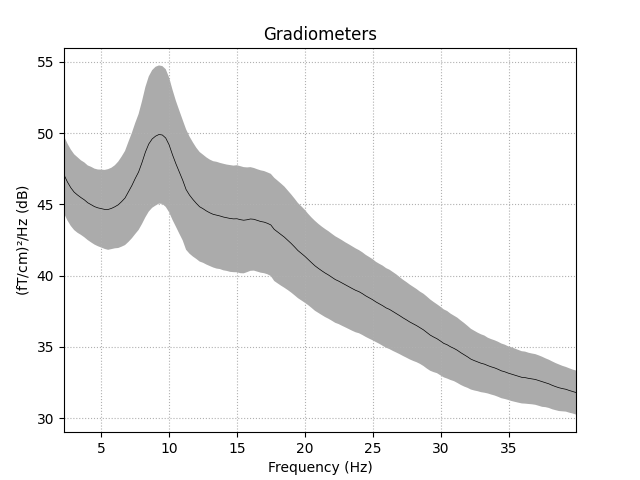Out:

Using multitaper spectrum estimation with 7 DPSS windows


Now let’s take a look at the spatial distributions of the PSD.

epochs.plot_psd_topomap(ch_type='grad', normalize=True)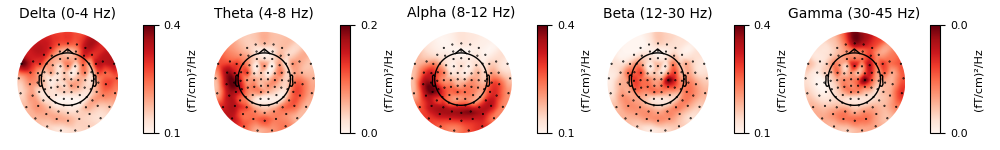Out:

Using multitaper spectrum estimation with 7 DPSS windows


Alternatively, you can also create PSDs from Epochs objects with functions that start with psd_ such as mne.time_frequency.psd_multitaper() and mne.time_frequency.psd_welch().

f, ax = plt.subplots()
psds, freqs = psd_multitaper(epochs, fmin=2, fmax=40, n_jobs=1)
psds = 10. * np.log10(psds)
psds_mean = psds.mean(0).mean(0)
psds_std = psds.mean(0).std(0)

ax.plot(freqs, psds_mean, color='k')
ax.fill_between(freqs, psds_mean - psds_std, psds_mean + psds_std,
color='k', alpha=.5)
ylabel='Power Spectral Density (dB)')
plt.show()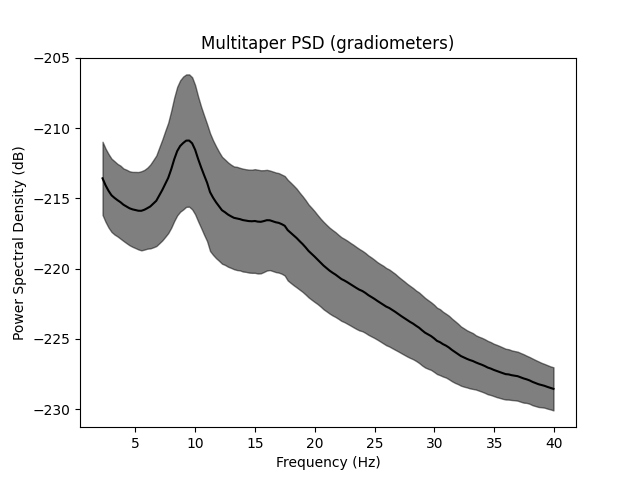Out:

Using multitaper spectrum estimation with 7 DPSS windows


Notably, mne.time_frequency.psd_welch() supports the keyword argument average, which specifies how to estimate the PSD based on the individual windowed segments. The default is average='mean', which simply calculates the arithmetic mean across segments. Specifying average='median', in contrast, returns the PSD based on the median of the segments (corrected for bias relative to the mean), which is a more robust measure.

# Estimate PSDs based on "mean" and "median" averaging for comparison.
kwargs = dict(fmin=2, fmax=40, n_jobs=1)
psds_welch_mean, freqs_mean = psd_welch(epochs, average='mean', **kwargs)
psds_welch_median, freqs_median = psd_welch(epochs, average='median', **kwargs)

# Convert power to dB scale.
psds_welch_mean = 10 * np.log10(psds_welch_mean)
psds_welch_median = 10 * np.log10(psds_welch_median)

# We will only plot the PSD for a single sensor in the first epoch.
ch_name = 'MEG 0122'
ch_idx = epochs.info['ch_names'].index(ch_name)
epo_idx = 0

_, ax = plt.subplots()
ax.plot(freqs_mean, psds_welch_mean[epo_idx, ch_idx, :], color='k',
ls='-', label='mean of segments')
ax.plot(freqs_median, psds_welch_median[epo_idx, ch_idx, :], color='k',
ls='--', label='median of segments')

ax.set(title='Welch PSD ({}, Epoch {})'.format(ch_name, epo_idx),
xlabel='Frequency (Hz)', ylabel='Power Spectral Density (dB)')
ax.legend(loc='upper right')
plt.show()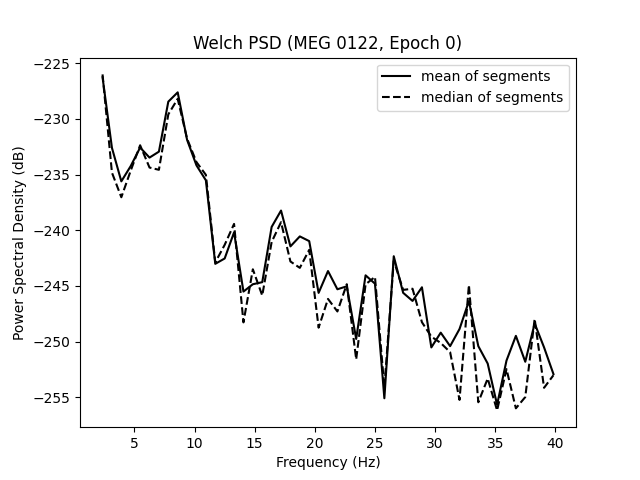Out:

Effective window size : 1.280 (s)
Effective window size : 1.280 (s)


Lastly, we can also retrieve the unaggregated segments by passing average=None to mne.time_frequency.psd_welch(). The dimensions of the returned array are (n_epochs, n_sensors, n_freqs, n_segments).

psds_welch_unagg, freqs_unagg = psd_welch(epochs, average=None, **kwargs)
print(psds_welch_unagg.shape)


Out:

Effective window size : 1.280 (s)
(108, 204, 49, 3)


## Time-frequency analysis: power and inter-trial coherence¶

We now compute time-frequency representations (TFRs) from our Epochs. We’ll look at power and inter-trial coherence (ITC).

To this we’ll use the function mne.time_frequency.tfr_morlet() but you can also use mne.time_frequency.tfr_multitaper() or mne.time_frequency.tfr_stockwell().

# define frequencies of interest (log-spaced)
freqs = np.logspace(*np.log10([6, 35]), num=8)
n_cycles = freqs / 2.  # different number of cycle per frequency
power, itc = tfr_morlet(epochs, freqs=freqs, n_cycles=n_cycles, use_fft=True,
return_itc=True, decim=3, n_jobs=1)


## Inspect power¶

Note

The generated figures are interactive. In the topo you can click on an image to visualize the data for one sensor. You can also select a portion in the time-frequency plane to obtain a topomap for a certain time-frequency region.

power.plot_topo(baseline=(-0.5, 0), mode='logratio', title='Average power')
power.plot(, baseline=(-0.5, 0), mode='logratio', title=power.ch_names)

fig, axis = plt.subplots(1, 2, figsize=(7, 4))
baseline=(-0.5, 0), mode='logratio', axes=axis,
title='Alpha', show=False)
baseline=(-0.5, 0), mode='logratio', axes=axis,
title='Beta', show=False)
mne.viz.tight_layout()
plt.show()

•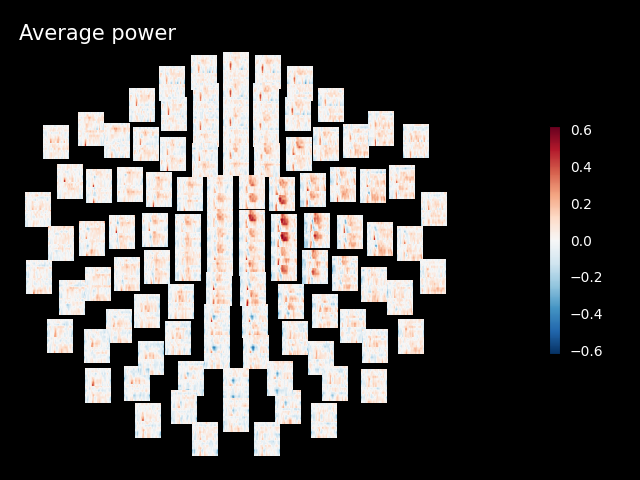•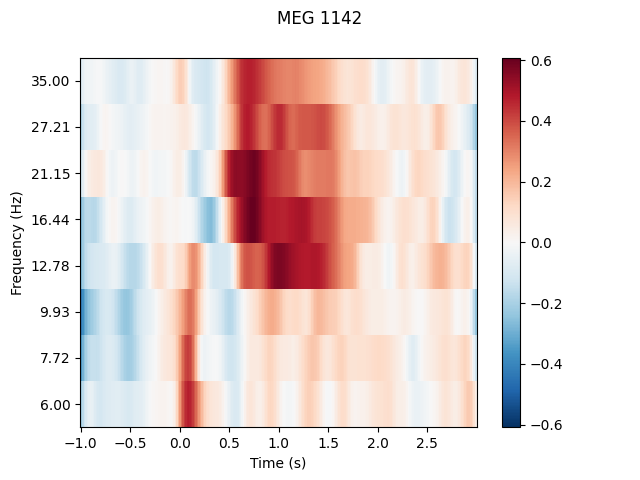•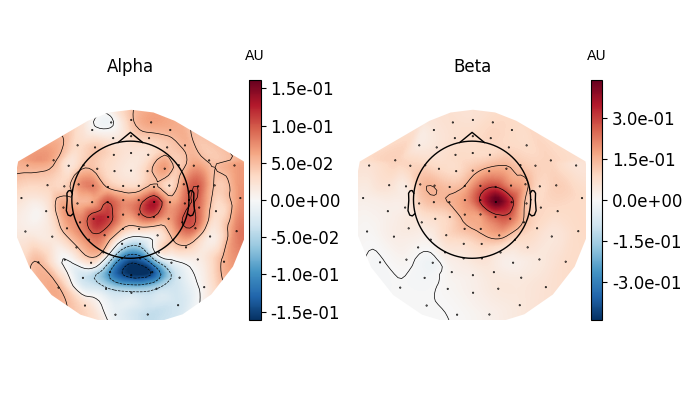Out:

Applying baseline correction (mode: logratio)
Applying baseline correction (mode: logratio)
Applying baseline correction (mode: logratio)
Applying baseline correction (mode: logratio)


## Joint Plot¶

You can also create a joint plot showing both the aggregated TFR across channels and topomaps at specific times and frequencies to obtain a quick overview regarding oscillatory effects across time and space.

power.plot_joint(baseline=(-0.5, 0), mode='mean', tmin=-.5, tmax=2,
timefreqs=[(.5, 10), (1.3, 8)])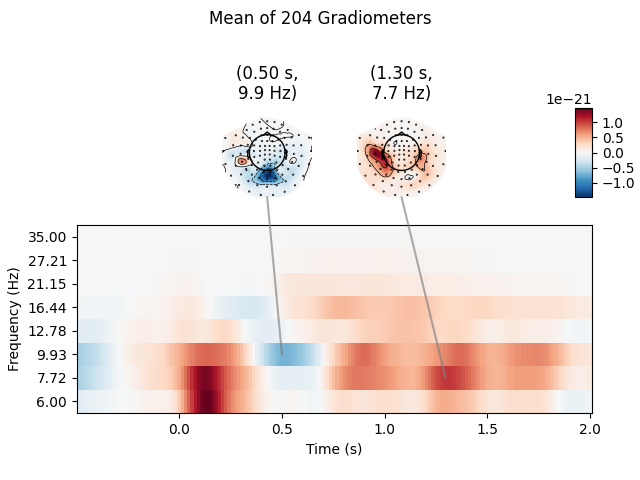Out:

Applying baseline correction (mode: mean)
Applying baseline correction (mode: mean)
Applying baseline correction (mode: mean)


## Inspect ITC¶

itc.plot_topo(title='Inter-Trial coherence', vmin=0., vmax=1., cmap='Reds')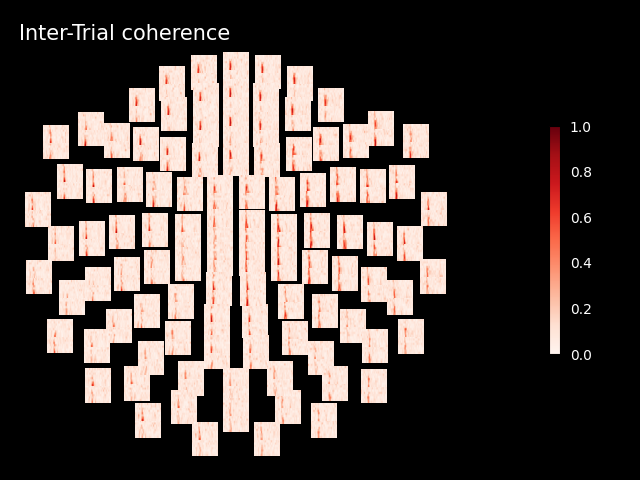Out:

No baseline correction applied


Note

Baseline correction can be applied to power or done in plots. To illustrate the baseline correction in plots, the next line is commented power.apply_baseline(baseline=(-0.5, 0), mode=’logratio’)

## Exercise¶

• Visualize the inter-trial coherence values as topomaps as done with power.

Total running time of the script: ( 0 minutes 57.095 seconds)

Estimated memory usage: 607 MB

Gallery generated by Sphinx-Gallery